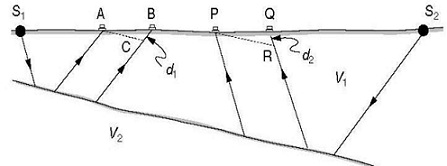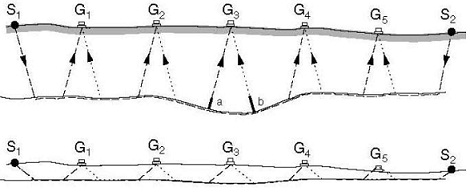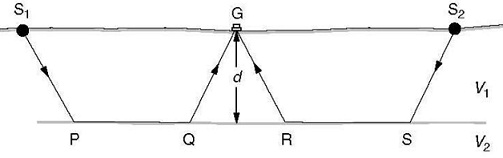#### Field Interpretation, Physics tutorial

Introduction:

Interpretation is necessary part of refraction fieldwork as success of survey depends on parameters like line orientation, shot positions geophone spacing, and spread lengths, that can be differed almost at will. Only if analysis keeps rate with data collection will right choices be made. Field interpretation has been made simpler by computer programs which can be implemented on portable PCs or on seismographs but such programs are based on very easy models and are not alternate for actually data.

Intercept Times:

Intercept time ti is stated as time at which back-extrapolated refracted arrival line cuts time axis. For a single refractor, it is connected to velocities and refractor depth by equation:

ti = 2d√(V22 -V12)/V1V2 = 2d/V1,2

The quantity V1,2 is stated by this equation. It has units of velocity and is about equal to V1 if V2 is very much larger than V1. Critical angle is then approximately 900 and delay suffered by refracted ray in travelling between surface and refractor is close to double vertical travel time. If difference between V1 and V2 is small, V1,2 can be very large. Intercept times are usually achieved by drawing best-fit lines through refracted arrival times but even very good fit is no guarantee that depth of refractor doesn't change in region near shot point, from which no refractions are seen. If, though, a long shot is utilized, there must be constant difference between long-shot and short-shot arrival times at points towards far end of the spread.Paths for energy travelling to geophones from S1 and S2 via lower refractor are identical from point B onwards. The intercept time can then be achieved by subtracting difference from long-shot arrival time at the short-shot location, and this can be completed exactly if there is geophone in this position when long shot is fired. Or else, use of nearest long-shot arrival at least decreases distance over which extrapolation should be made.

Multiple Layers:

Intercept-time equation can be extended to cases involving number of vitally refracting layers. If intercept time related with nth refractor is tn, then:

n = 2d1/V1,n+1 + 2d2/V2,n+1 · · ·+· · · 2dn/Vn,n+1

Where dn is thickness of nth layer, that overlies nth refracting interface, at which velocity increases from Vn to Vn+1. Definition of different quantities Vm,n is exactly analogous to definition of V1,2 given above. Presence of intermediate layers may be identified by comparing long- and short-shot data, but at least two points are required to describe velocity and three for any confidence to be placed in estimate. At best, therefore, only four layers can be easily investigated with a 12-channel system.

Effect of Dip:

Depths evaluated from refraction surveys are connected to geophone and shot-point elevations that should thus be estimated to attain true picture of subsurface refractor. In addition, depths determined are perpendicular, not vertical, distances to interfaces from shot points or geophones. Horizontal formulae can be applied without alteration wherever ground surface and refractor are parallel. More generally their slopes will be different.

The intercept-time equations need true value of V2 to be utilized. Though, wave which travels down-dip not only has to travel further at velocity V2 to reach more remote geophones, but also further at slow velocity V1 in upper layer. It thus arrives late, with low apparent velocity. Reverse is true shooting up-dip, when arrivals at further geophones may essentially precede those at nearer ones. Slope of line through refracted arrivals on time-distance plot depends on dip angle, α, according to:

Vapp = V2/(1 + sinα)

If shots are fired from both ends of spread, different apparent velocities will be estimated as sign of dip angle will vary. For dips of less than about 100, true velocity is provided by dip-velocity equation:

2/V2 = 1/Vup + 1/Vdown

Refractor Relief and True Velocities:

Most refractors, except water table, are irregular. If there were only single local depression in the otherwise flat refractor, refracted arrivals from shots in opposite directions would plot on straight lines of equal slope, and differences between two arrival times at each geophone would plot on the line with double this slope. Exception to this rule would appear to be geophone immediately above depression.Refracted energy from S1 arrives later at B than at A not only due to greater distance travelled along refractor but also due to extra distance d1 travelled in low velocity layer. Energy from S2 arrives earlier at P than would be forecasted from time of arrival at Q, by time taken to travel d2 at velocity V1. Lines AC and PR are parallel to refractor. Though, both waves would arrive late at this point and, for small dips; delays would be extremely similar. Difference between arrival times would therefore be almost same as if no depression existed, plotting on straight line produced by horizontal parts of interface. Argument can be extended to the refractor with series of depressions and highs. Given that dip angles are low, difference points will plot along straight line with slope corresponding to half the refractor velocity. Where the refractor has constant dip, slope of the difference line will equivalent to dip velocity equation. Sum of slopes of individual lines, giving the graphical expression:Arrivals at G3 of energy from S1 and S2 are delayed by approximately same amounts 'a' and 'b'). The significance of long shots is clear, as part of spread over which first arrivals from short shots at both ends have come via refractor is probable to be rather short and may not even exist. It is even at times possible, particularly when centre shots have been utilized, for differencing method to be applied to intermediate refractor. They are plotted using the arbitrary time zero line placed where it will cause least confusion with other data.

Reciprocal Time Interpretation:

Reciprocal time, tR, is stated as time taken for seismic energy to travel between two long-shot positions. Difference between tR and sum of travel times tA and tB from two long-shots to any given geophone, G, is:

tA + tB - tR = 2D/F

Here D is depth of refractor beneath G and F is a depth conversion factor. If there is only a single interface, D is equivalent to thickness, d, of upper layer and F is equivalent to V1,2. If there are more interfaces, F is composite of all velocities involved, weighted according to layer thicknesses. At short shots 2D/F = ti (intercept time) and F values can be computed. Ways in which F differs between the points may be very difficult, but linear interpolation is generally enough in field.Sum of travel times from S1 and S2 to G varies from reciprocal time, tR, taken to travel from S1 to S2 by difference between times taken to travel QR at velocity V2 and QGR at velocity V1.

Though tR can be estimated directly, it is more suitable to compute it from equation above using intercept times. This can be done given that geophones are located at short-shot points when long shots are fired (so that tA + tB at those points can be estimated). Estimates of tR made using data from two ends must agree within limits of error of method (i.e. within 1-2 ms-1). If they are not, raw data and calculations must be thoroughly checked to find reason for discrepancy. Short-shot reciprocal times are estimated directly if short-shots are fired at end-geophone positions, and fact that they must be equal may help in picking arrivals. Though, they have little interpretational importance.

Tutorsglobe: A way to secure high grade in your curriculum (Online Tutoring)